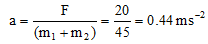# First Law Of Motion By Help Of Second Law Of Motion

## First Law Of Motion By Help Of  Second  Law Of Motion

According to first law of motion, if there is no force, there is no change in state of rest or of uniform motion. In other words, if a force is applied, it may change the state of rest or of uniform motion. If the force is not sufficient, if may not produce a change but only try to do so. Hence force is that which changes o tries to change the state of rest or of uniform motion of a body in straight line.

Hence we get the definition of force from Newton’s first law of motion.

Newton’s first law of motion can be deduced from Newton’s’ second law of motion.

According to second law of motion,

F = ma

if F = 0, then a = 0

Since m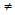0

So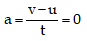or mv = mu

or v - u = 0 or v = u

or v = u [after more time]

Which means that the velocity of the body cannot change in absence of external force. If the body is initially at rest i.e., if u = 0, v = 0 and if u = 5 ms-1, v = 5ms-1.

Thus, it follows that a body will continue to be in the state of rest or of uniform motion along a straight line if no external force acts on it and this is the first law. thus, first law can be deduced from second law of motion.

Question: A force F1 acting on a body of 2 kg produces an acceleration of 2.5 ms2. Another force F2 acting on the another body of mass 5 kg produces an acceleration of 2 m/sec2. Find the ratio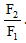.

Solution: For fist body F = ma

F1 = 2 × 2.5 = 5N

For second body F2 = 5 × 2 = 10N

So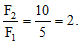Question. A force of 20N acting on amass m1 produces an acceleration of 4 ms-2. The same force is applied on mass m2 then the acceleration produced is 0.5 ms-2. What acceleration would the same force produce, when both masses are tied together ?

Solution: For mass m1 : F = 20N, a = 4 ms-2

then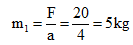For mass m2 : F = 20N, a = 0.5 ms-2

then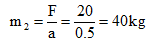When m2 and m2 are tied together :

Total mass = m1 + m2 = 45 kg. F = 20N

then